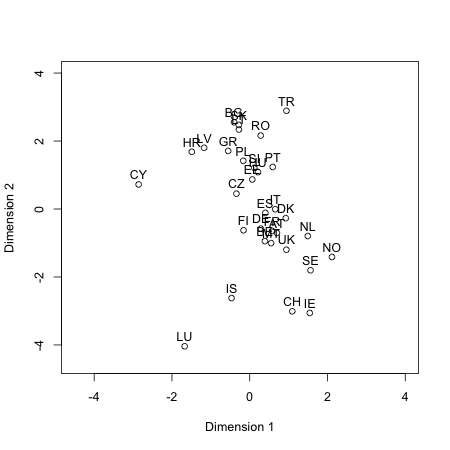Visualizing Asymmetry

Introduction

Asymmetric matrices are square, and have the same number of rows and columns, which refer to the same set of objects. At least some elements in the upper triangle are different from the corresponding elements in the lower triangle. That is, we have for the asymmetric matrix $$Q$$ the identity $$Q \neq Q^T$$, where $$Q^T$$. denotes the transpose of the matrix $$Q$$ An example of an asymmetric matrix is a migration table. In this example, the rows and columns refer to the same countries. For example, the countries in the rows are the home countries, the columns are the destination countries. These tables can be used to answer two questions. The first question is which countries are similar. For instance, similar countries exchange more students than dissimilar countries because of a cultural or other similarity. The second question concerns which countries are more successful in attracting students. The following script generates data from the Erasmus student exchange program to work with. To keep the results of the analysis readable we work in this example with five countries.

library("asymmetry")
data("studentmigration")
idx <- c(3,4,25,27,31) #select five countries
studentmigration[idx,idx]
##     CZ  DK  FI  UK  TR
## CZ   0 190 420 582 230
## DK  42   0  28 650 119
## FI 181 106   0 643  48
## UK 186 244 228   0  95
## TR 615 232 143 617   0

The data give the number of inbound and outbound students in the Erasmus program. These migration data of students participating in the Erasmus program may give insight in the similarity between countries and the attractiveness of countries. The Erasmus program is a student exchange program from the European Union. Three million students had taken part since the start of the program in 1987. To join this program a student has study at least three months or do an internship of at least two months in another country. The entries in the table are as follows: 190 students move from the Czech Republic (CZ) to Denmark (DK), whereas 42 students move from Denmark to the Czech Republic. The complete table lists the home and destination country of 268.142 students in the academic year 2012- 2013.

Decomposition of an asymmetric matrix

The decomposition of an asymmetric matrix into a symmetric matrix and a skew-symmetric matrix is an elementary result from mathematics that is the cornerstone of this package. The decomposition into a skew-symmetric and a symmetric component is written as: $Q = S + A,$ where $$S$$ is a symmetric matrix with averages $$(q_{ij}+q_{ji})/2$$, and $$A$$ is a skew-symmetric matrix with elements $$(q_{ij}-q_{ji})/2$$. A square matrix is skew-symmetric if the transpose can be obtained by multiplying the elements of the matrix by minus one, that is $$A^T = -A$$. Another, perhaps more convenient way to state this property is $$a_{ij}=-a_{ji}$$, that is, if we interchange the subscripts the sign changes. It follows that the diagonal elements $$a_{ii}$$ of a skew-symmetric matrix are zero.

The skew symmetric part $$A$$ of a portion of the data is generated by the following script

q1 <- skewsymmetry(studentmigration[idx,idx])
q1$A ## CZ DK FI UK TR ## CZ 0.0 74.0 119.5 198.0 -192.5 ## DK -74.0 0.0 -39.0 203.0 -56.5 ## FI -119.5 39.0 0.0 207.5 -47.5 ## UK -198.0 -203.0 -207.5 0.0 -261.0 ## TR 192.5 56.5 47.5 261.0 0.0 Similarly, the symmetric part is obtained by q1$S
##       CZ    DK    FI    UK    TR
## CZ   0.0 116.0 300.5 384.0 422.5
## DK 116.0   0.0  67.0 447.0 175.5
## FI 300.5  67.0   0.0 435.5  95.5
## UK 384.0 447.0 435.5   0.0 356.0
## TR 422.5 175.5  95.5 356.0   0.0

The decomposition is additive, and because the two components $$S$$ and $$A$$ are orthogonal, the decomposition of the sum of squares of the two matrices is also additive. Because the sum of the cross products vanishes, the sum of squares consists of two components

$\sum_{i=1}^n\sum_{j=1}^n q_{ij}^2 = \sum_{i=1}^n\sum_{j=1}^n s_{ij}^2 + \sum_{i=1}^n\sum_{j=1}^n a_{ij}^2.$

The summary method provides the sum of squares due to symmetry and the sum of squares due to skew-symmetry.

summary(q1)
##                     SSQ   Percent
## Symmetry      1980666.5  79.49979
## Skew-symmetry  510744.5  20.50021
## Total         2491411.0 100.00000

The additivity of the two sums of squares provides a justification for analyzing the two components independently. For instance, the symmetric part can be represented by a symmetric method such as multidimensional scaling or hierarchical cluster analysis. Suggestions for the analysis of the skew-symmetric part are the heatmap, the linear model or the Gower diagram. In a later stage the results of these analyses of the two components can possibly be used to suggest a joint model the table $$Q$$. The results of a hierarchical cluster analysis are shown below.

clus <- hclust(as.dist(1/q1$S)) plot(clus,xlab=NA,sub=NA)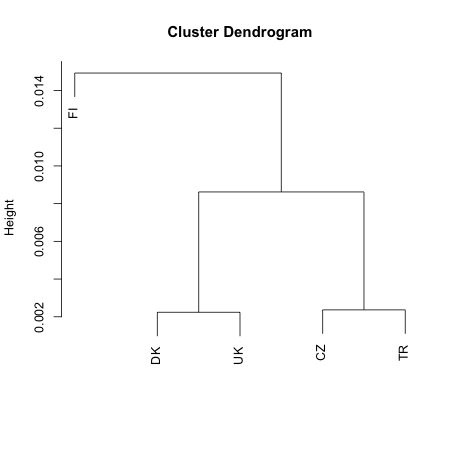The linear model provides a useful summary of the skew-symmetric matrix. This model is based on the difference of the scale values $$c_i$$ of two objects, and is written as $a_{ij}=c_i - c_j.$ It is easily seen that this model is skew-symmetric because we have $$a_{ji} = c_j - c_i = -(c_i - c_j) = -a_{ji}.$$ Let $c_j = {1 \over n} \sum_{i=1}^n a_{ij}$ denote the average of a column of this matrix. This estimate minimizes the sum of squares loss function, and is therefore a least-squares estimate. There is an indeterminacy in the model, because $$\tilde{c_i} = c_i + d$$, where $$d$$ is any number is also a solution with the same least-squares loss. Therefore, the identification constraint $$\sum_{i=1}^n c_i = 0$$ is used. An example is given by the following line of code. q1$linear
##     CZ     DK     FI     UK     TR
##   39.8    6.7   15.9 -173.9  111.5

Inserting the definition of skew-symmetry in this estimate, that is $c_j = {1 \over n} \sum_{i=1}^n a_{ij} = {1 \over (2n)} \sum_{i=1}^n ( q_{ij} - q_{ji} )$ we see that this estimate is equal to $${1 \over 2}$$ the difference of the column mean and the row mean

Heatmap

Color is widely used in data visualization to show data values. A heatmap displays values in a data matrix by colors and reorders the rows and columns of this matrix by dendograms. The heatmap function hmap is a quick way to visualize skew-symmetric data. The order of the rows and columns is given by the row sums of the matrix, and not by a dendogram as in a usual heatmap. A permutation of the rows and columns is derived from the number of positive elements in a row of the matrix. If the matrix has no circular triads all values in the upper triangle are positive and all values in the lower triangle are negative. This method can display the signs or values of the elements in the matrix. The option dominance gives the signs of the skew-symmetric matrix, otherwise the values are shown.

library(RColorBrewer)
# creates a color palette from red to blue
my_palette <- colorRampPalette(c("red", "white", "blue"))(n = 299)
col_breaks = c(seq(-4000,-.001,length=100),  # negative values are red
seq(-.001,0.01,length=100),                # zeroes are white
seq(0.01,4000,length=100))                 # positive values are blue

hmap(q1, col = my_palette)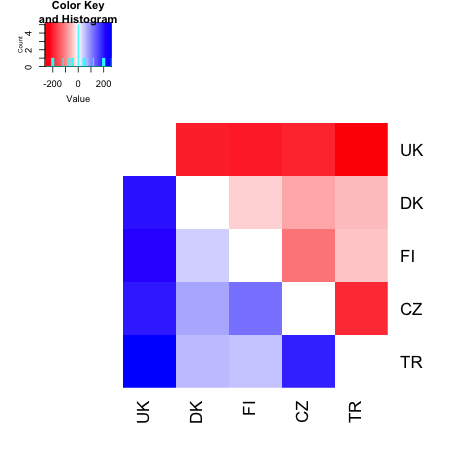Blue values correspond to positive values, whereas red values correspond to negative values. The intensity of the colors show the magnitude. Because the values in the column UK are blue, which point to positive net migration, the UK attracts more students from abroad than any other country. In this example, the UK is the most popular destination for international students. The second most popular country is Denmark (DK), followed by Finland and the Czech republic (CZ). The least popular country of these five countries is Turkey. By permuting the rows and columns the data in this order the values in the upper triangle are red, and the values in the lower triangle are blue, corresponding to negative and positive values respectively in the data matrix.

Heatmap application: finding circular triads

data(studentmigration)
idx <- c(18,22,27,2,13,31) #select 6 countries
q1 <- skewsymmetry(studentmigration[idx,idx])
q1$A ## NL RO UK BG LV TR ## NL 0.0 -43.0 492.0 -7.0 -25.5 -39.5 ## RO 43.0 0.0 51.0 -3.0 1.5 -109.5 ## UK -492.0 -51.0 0.0 -49.5 -23.0 -261.0 ## BG 7.0 3.0 49.5 0.0 -15.0 27.5 ## LV 25.5 -1.5 23.0 15.0 0.0 -60.5 ## TR 39.5 109.5 261.0 -27.5 60.5 0.0 A heatmap of this skew-symmetric table is generated by the following script # creates a color palette from red to blue my_palette <- colorRampPalette(c("red", "white", "blue"))(n = 299) col_breaks = c(seq(-4000,-.001,length=100), # negative values are red seq(-.001,0.01,length=100), # zeroes are white seq(0.01,4000,length=100)) # positive values are blue data(studentmigration) hmap(studentmigration[idx,idx], dominance = FALSE, col = my_palette, key = FALSE, xlab = "Destination country", ylab = "Home country", colsep = c(1:6), rowsep = c(1:6))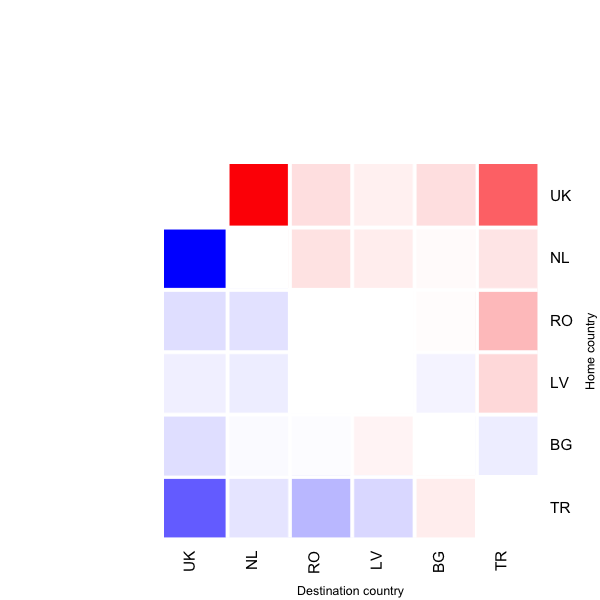In this heatmap some elements in the upper triangle are blue and some are red, which means that no ordering can give a satisfactory account of the skew-symmetries and that circular triads are present. In this table we find a circular triad between Turkey, Latvia, and Bulgaria. There are more Turkish students migrating to Latvia then there are Latvian students migrating to Turkey, there are more Latvian students moving to Bulgaria than there are Bulgarian students moving to Latvia, and finally more Bulgarian students move to Turkey than there are movements in the other direction. Slide-vector model The slide vector model is a multidimensional scaling (MDS) model for asymmetric data. MDS fits symmetric distances to data, whereas this model fits modified distances which are asymmetric. A distance model is fitted to the symmetric part of the data whereas the asymmetric part of the data is represented by projections of the coordinates onto the slide-vector. The slide-vector points in the direction of large asymmetries in the data. The distance is modified in such a way that the distance between two points that are parallel to the slide-vector is larger in the direction of this vector. The distance is smaller in the opposite direction. If the line connecting two points is perpendicular to the slide-vector the difference between the two projections is zero. In this case the distance between the two points is symmetric. The algorithm for fitting this model is derived from the majorization approach to multidimensional scaling. The slide-vector model is given by the following equation $d_{ij}(X;z)=\sqrt{\sum_{s=1}^p(x_{is}-x_{js}+z_{is})^2}.$ The squared distances can be decomposed in a linear skew-symmetric and symmetric part. $d_{ij}^2(X;z)=\sum_{s=1}^p (x_{is}-x_{js})^2+\sum_{s=1}^p z_{is}^2 + 2\sum_{s=1}^p( x_{is}-x_{js})z_{is}.$ The following lines of code generate a two-dimensional representation of the English towns data for the slide-vector model. data(Englishtowns) v<-slidevector(Englishtowns, ndim = 2, itmax = 2500, eps = .0000001, verbose = FALSE) plot(v,col="blue",ylim=c(-300,300),xlim=c(-300,300))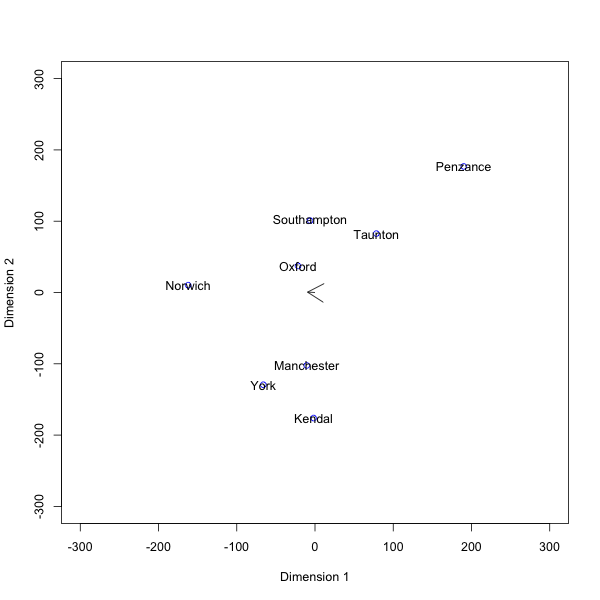A decomposition of the residuals can be obtained using the following lines of code q2 <- skewsymmetry(v$resid)
summary(q2)
##                    SSQ   Percent
## Symmetry      1643.222  55.97993
## Skew-symmetry 1292.155  44.02007
## Total         2935.377 100.00000

MDS with unique dimensions

This MDS model has both common that are shared by all objects and unique dimensions that apply to one object and not to the other objects. The shared dimensions provide a Euclidean map of the objects in low-dimension space, whereas unique dimension apply to one object. A unique dimension has a non zero value for only one object, the coordinates for the other objects are zero. There are as many unique dimensions as there are objects. An asymmetric version of this model has two sets of unique dimensions: one for the rows and one for the columns. The distance in this model is defined as: $d_{ij}(X)=\sqrt{\sum_{s=1}^p (x_{is}-x_{js})^2 + r_{i}^{2}+c_{j}^{2}}.$

data("studentmigration")
mm<-studentmigration
mm[mm==0]<-.5          # replace zeroes by a small number
mm <- -log(mm/sum(mm)) # convert similarities to dissimilarities
v<-mdsunique(mm, ndim = 2, itmax = 2100, verbose=FALSE, eps = .0000000001)
plot(v, yplus = .3, ylim = c(-4.5, 4), xlim = c(-4.5, 4))